# How to linearize max, min, and abs functions

In this post you will see how to linearize max functions, min functions, and absolute value functions. These can be expressed mathematically as: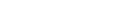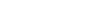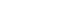# Linearization of the product of two variables

Often when writing a model, the most straightforward way of writing a constraint is by multiplying two variables. Then, in order to solve the model we need to linearize it. There comes the problem, as I always have problems reminding how to linearize a product of variables.

# Linearizing the product of two binary variables

Suppose your model has the product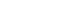, where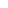,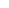and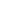are binary. There is an easy way of linearizing that equation. Add the three inequalities below